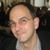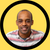# Discussion on: High order functionsHi Denis!

This looks awesome. I think all of your examples fall into the first category (functions that take functions as arguments), but your definition ("A function that takes another function as argument and/or return function as output") highlights there's also a second kind of higher order function, that returns functions as output, one possible example is `compose`, which probably looks something like this

``````compose = (f1, f2) => (x) =>  f1( f2(x) )
``````

Then your `g(f(x))` example from the beginning is just `compose(g,f)`, and you can continue abstracting, and compose composed functions - like

``````f = (x) => x + 3
g = (x) => 4 * x
compose( compose(g,g), compose(f,g) )(4)
304
``````

Another example would be `repeat` which would take a function, and compose it on itself a number n times, like

`````` repeat = (f,n) => ((n == 1) ? f : compose(f, repeat(f, n -1)))
``````

I'm not sure if that kind of higher order function is built into javascript already?Denis Woldemariam

No I don't think so. Don't quote me though because I am still learning about it. I think you have a point. The point of the article was to introduce developers to the most used higher order functions in javaScript and that is the reason why I focused on the first kind. I should have given examples to the second as well but like you said I am not sure if we have those in javaScript. I will try to do some more research and see if I can give examples to that. Thank you for the feedbackYeah - I think I did a little looking around after I asked that question (MDN has a section on closures that's relevant), and it looks like there are performance reasons to not create functions at runtime the way I was showing - the JS runtime is able to do some optimizations when the functions are expressed in the code - and less able to do that when they're created in memory from a function call (like my `compose` example).